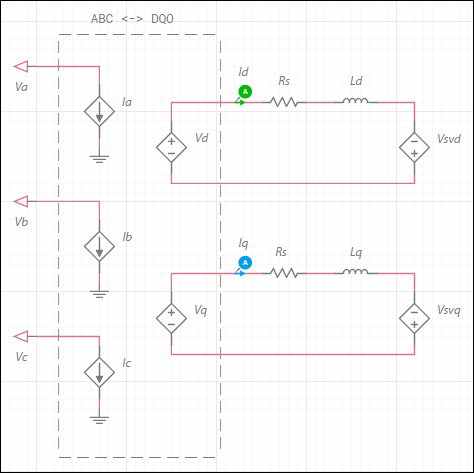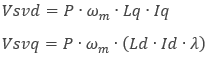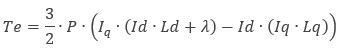# Synchronous permanent magnet model

Model assumptions

• Uniform air-gap
• No slot harmonics
• No stator saturation
• Sinusoidally distributed windings
• No zero phase sequence (system is balanced)

Configuration of the machine electrical systemwhere

= number of poles
ωm = angular speed
λ = flux linkage induced by the permanent magnet

The elements in the dashed region represent the bidirectional ABC↔DQ0 coordinate transform in the rotor reference frame. Refer to the DQ0 coordinate transforms section for representative equations.

The generated electromagnetic torque, Te, is:Parameter Description
d-axis inductance Combined leakage inductance and magnetizing inductance on d-axis. Ld in the generated electromagnetic torque equation.
q-axis inductance Combined leakage inductance and magnetizing inductance on q-axis. Lq in the generated electromagnetic torque equation.
Stator resistance Rs in the electrical diagram.
Magnet flux Flux linkage induced by the permanent magnet. λ in the equations for Vsvq and Te.
Number of pole pairs P in the equations for Vsvd, Vsvq and Te.
Shaft inertia J<sub>rotor</sub> on the machine model diagram in <a href="/help/components/machine-modeling/">Machine modeling</a>.
Shaft friction This is F<sub>rotor</sub> on the machine model diagram in <a href="/help/components/machine-modeling/">Machine modeling</a>.
Initial angular speed Rotational measurement of the shaft angle in rad/s at the start of the simulation.
Initial angle Initial shaft angle in radians.